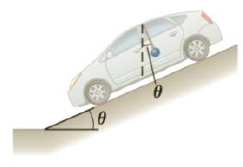Chapter 4, Problem 87AP

Chapter
Section
Textbook Problem

A car accelerates down a hill (Fig. P4.87), going from rest to 30.0 m/s in 6.00 s. During the acceleration, a toy (m = 0.100 kg) hangs by a string from the car’s ceiling. The acceleration is such that the string remains perpendicular to the ceiling. Determine (a) the angle θ and (b) the tension in the string.Figure P4.87

(a)

To determine
The angle of incline.

Explanation

Given info: A car accelerates from rest to 30 m/s in 6.00 s. Mass of the toy is 0.100 kg.

The free body diagram is given below.

• T is the tension.
• m is the mass of the toy.
• g is the acceleration due to gravity.
• a is the acceleration.

From the diagram,

mgsinθ=ma

On Re-arranging, the angle of incline is,

θ=sin1(ag)                                               (I)

The formula for acceleration is,

a=vut                                                      (II)

• v is the final velocity.
• u is the initial velocity

(b)

To determine
The tension in the string.

Still sussing out bartleby?

Check out a sample textbook solution.

See a sample solution

The Solution to Your Study Problems

Bartleby provides explanations to thousands of textbook problems written by our experts, many with advanced degrees!

Get Started

Find more solutions based on key concepts